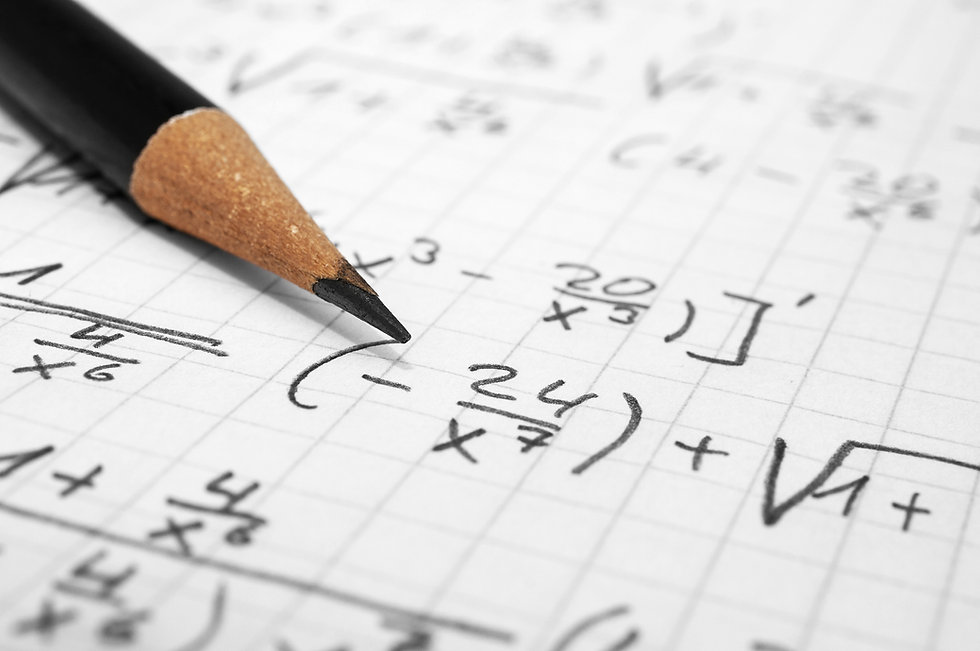# CHARTER SCHOOL## 1 credit

This course includes the following topics commonly found in pre-calculus mathematics:
trigonometry; sets and logical statements; functions, polynomial functions; logarithmic and
trigonometric functions (including circular functions); complex numbers, plane analytic geometry; matrices; vectors, induction, and the binomial function; permutations, combinations, and probability and elementary statistics; sequences, series and limits. It also includes derivatives of polynomials and curve sketching, and conic sections. This course reviews and unifies mathematical experience and acts as a transition from the mathematics commonly associated with the secondary schools to higher mathematics.